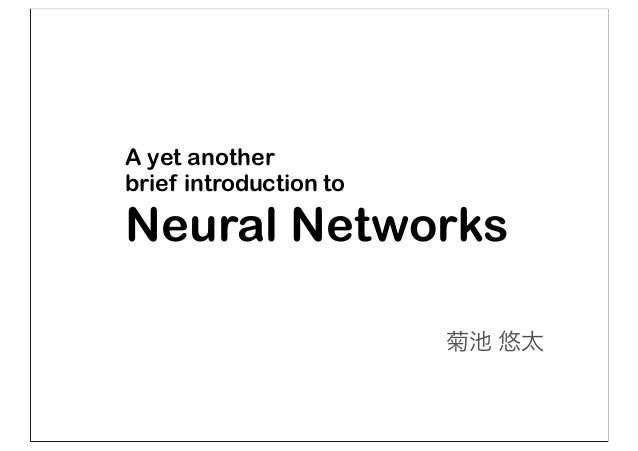Successfully reported this slideshow.
We use your LinkedIn profile and activity data to personalize ads and to show you more relevant ads. You can change your ad preferences anytime.Upcoming SlideShare
Loading in …5
×

# A yet another brief introduction to neural networks

12,836 views

Published on

Published in: Technology
• Full Name
Comment goes here.

Are you sure you want to Yes No
Your message goes here• Be the first to comment

### A yet another brief introduction to neural networks

1. 1. A yet another brief introduction to Neural Networks 菊池 悠太
2. 2. Neural Network 入力層x 隠れ層z 出力層y
3. 3. 入力層x 隠れ層z 出力層y 生データ，抽出した素性 予測 Neural Network
4. 4. 入力層x 隠れ層z 出力層y 例えば，手書き数字認識 784次元 10次元 MNIST (28*28の画像) ３！！ [0.05, 0.05, 0.05, 0.40, 0.05, 0.05, 0.15, 0.05, 0.15, 0.05] 10次元の確率分布 （左から，入力画像が，   0である確率，    1である確率    ...    9である確率） 28*28= 784次元の数値ベクトル
5. 5. 入力層x 隠れ層z 出力層y Neuron 隠れユニットjの 入力層に対する重み W1 隠れユニットj
6. 6. 隠れユニットjの 入力層に対する重み W1 隠れユニットj 出力ユニットk 出力ユニットkの 隠れ層に対する重みW2 入力層x 隠れ層z 出力層y Neuron
7. 7. 入力層x 隠れ層z 出力層y W1 W2 行列で表現 層間の重みを行列で表現
8. 8. Neural Networkの処理 - Forward propagation - Back propagation - Parameter update
9. 9. Neural Networkの処理 - Forward propagation - Back propagation - Parameter update
10. 10. Forward Propagation 入力層x 隠れ層z 出力層y 入力に対し出力を出す output x input y
11. 11. 入力層x 隠れ層z 出力層y z = f(W1x + b1) 入力層から隠れ層への情報の伝播 非線形活性化関数f( ) tanh とか sigmoid とか f(x0) f(x1) f(x2) f(x3) f(x) =
12. 12. 入力層x 隠れ層z 出力層y z = f(W1x + b1) 入力層から隠れ層への情報の伝播 非線形活性化関数f( ) tanh,sigmoid reLU, maxout... f(x0) f(x1) f(x2) f(x3) f(x) = f( )
13. 13. 入力層x 隠れ層z 出力層y z = f(W1x + b1) f( ) 入力層から隠れ層への情報の伝播 ⼊入⼒力力の情報を 重み付きで受け取る 隠れユニットが出す 出⼒力力値が決まる
14. 14. 入力層x 隠れ層z 出力層y z = f(W1x + b1) 入力層から隠れ層への情報の伝播 f( ) 非線形活性化関数f( ) tanh,sigmoid reLU, maxout... f(x0) f(x1) f(x2) f(x3) f(x) =
15. 15. 入力層x 隠れ層z 出力層y 出⼒力力層⽤用の 非線形活性化関数σ( )    タスク依存 隠れ層から出力層への情報の伝播 y = (W2z + b2)
16. 16. 入力層x 隠れ層z 出力層y 出⼒力力層⽤用の 非線形活性化関数σ( )    タスク依存 隠れ層から出力層への情報の伝播 y = (W2z + b2)
17. 17. 入力層x 隠れ層z 出力層y タスク依存の出力層 解きたいタスクによって σが変わる - 回帰 - 二値分類 - 多値分類 - マルチラベリング y = (W2z + b2)
18. 18. 実数 入力層x 隠れ層z 出力層y 回帰のケース 出力に値域はいらない 恒等写像でそのまま出力 (a) = a y = (W2z + b2)
19. 19. [0:1] 二値分類のケース 出力層は確率 σは0.0~1.0であって欲しい (a) = 1 1+exp( a) Sigmoid関数入力層x 隠れ層z 出力層y y = (W2z + b2)
20. 20. 入力層x 隠れ層z 出力層y 多値分類のケース 出力は確率分布 各ノード0以上，総和が1 Softmax関数 sum( 0.2 0.7 0.1 )=1.0 (a) = exp(a) exp(a) y = (W2z + b2)
21. 21. 入力層x 隠れ層z 出力層y マルチラベリングのケース 各々が独立に二値分類 (a) = 1 1+exp( a) element-wiseで Sigmoid関数 [0:1] [0:1] [0:1] y = (W2z + b2)
22. 22. ちなみに多層になった場合 ... 出力層だけタスク依存 隠れ層はぜんぶ同じ 出力層 隠れ層1 隠れ層N ...
23. 23. Forward Propagation 入力層x 隠れ層z 出力層y z = f(W1x + b1) y = (W2z + b2)
24. 24. Neural Networkの処理 - Forward propagation - Back propagation - Parameter update
25. 25. Back Propagation 入力層x 隠れ層z 出力層y 正解t NNが入力に対する出力の 予測を間違えた場合 正解するように修正したい
26. 26. Back Propagation 入力層x 隠れ層z 出力層y 正解t NNが入力に対する出力の 予測を間違えた場合 正解するように修正したい 修正対象: 層間の重み z = f(W1x + b1) ↑と，バイアス y = (W2z + b2)
27. 27. Back Propagation 入力層x 隠れ層z 出力層y 正解t 誤差関数を最⼩小化するよう修正 E( ) = 1 2 y( ) t 2 E = K k=1 tk log yk E = t log y (1 t) log(1 y) E = K k=1 t log y + (1 t) log(1 y) いずれも予測と正解が 違うほど⼤大きくなる
28. 28. Back Propagation 入力層x 隠れ層z 出力層y 正解t  出力ラベルと正解の差 ノードの誤差を計算 y = y t
29. 29. Back Propagation 入力層x 隠れ層z 出力層y 正解t ノードの誤差を計算  出力ラベルと正解の差 自分が情報を伝えた先の 誤差が伝播してくる z = WT 2 yf (az) y = y t
30. 30. Back Propagation 入力層x 隠れ層z 出力層y 正解t y = y t z = WT 2 yf (az)  出力ラベルと正解の差 ノードの誤差を計算 自分の影響で上で発生した誤差
31. 31. Back Propagation 入力層x 隠れ層z 出力層y 正解t 重みの勾配を計算 ⾃自分が上に伝えた 情報で発⽣生した誤差 En W2 = yzT En W1 = zxT
32. 32. Back Propagation 入力層x 隠れ層z 出力層y 正解t 重みの勾配を計算 ⾃自分が上に伝えた 情報で発⽣生した誤差 En W2 = yzT En W1 = zxT
33. 33. Neural Networkの処理 - Forward propagation - Back propagation - Parameter update
34. 34. Update parameters 入力層x 隠れ層z 出力層y 正解t 重みの更新 W1 = W1 En W1 W2 = W2 En W2
35. 35. Update parameters 入力層x 隠れ層z 出力層y 正解t 重みの更新 W1 = W1 En W1 W2 = W2 En W2 -Gradient Descent -Stochastic Gradient Descent -SGD with mini-batch   修正するタイミングの違い
36. 36. Neural Network の処理まとめ
37. 37. Forward Propagation 入力層x 隠れ層z 出力層y output x input y z = f(W1x + b1) y = (W2z + b2) 入力から予測
38. 38. Back Propagation 入力層x 隠れ層z 出力層y 正解t 誤差と勾配を計算 z = WT 2 yf (az) y = y t En W2 = yzT En W1 = zxT
39. 39. Update parameters 入力層x 隠れ層z 出力層y 正解t 勾配方向へ重み更新 W1 = W1 En W1 W2 = W2 En W2
40. 40. ちなみにAutoencoder Neural Networkの特殊系 1. 入力と出力の次元が同じ 2. 教師信号が入力そのもの 入力を圧縮※1して復元 ※1 圧縮(隠れ層が入力層より少ない)でなくても，適切に正則化すればうまくいく
41. 41. Neural Networkの特殊系 Autoencoder z = f(W1x + b1) y = (W2z + b2)
42. 42. Neural Networkの特殊系 Autoencoder y = (W2z + b2) (a) = 1 1+exp( a) element-wiseで Sigmoid関数 マルチラベリングのケースに該当 画像の場合，各画素(ユニット)ごとに 明るさ(0.0:黒, 1.0:白)を判定するため
43. 43. Autoencoderの学習するもの
44. 44. Denoising Autoencoder add noise denoise 正則化法の一つ，再構築+ノイズの除去